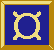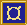# ¤ Point, Line, et al

Geometry, part 1

## Types

```data Point = Point {xcoord :: Int, ycoord :: Int}
instance Num Point
instance Move Point
instance Ix Point
instance Eq Point
instance Ord Point
instance Show Point

data Line = Line Point Point
instance Move Line
instance Eq Line
instance Ord Line
instance Show Line

data Rect = Rect {rectpos :: Point, rectsize :: Size}
instance Move Rect
instance Eq Rect
instance Ord Rect
instance Show Rect

type Size = Point

pP :: Int -> Int -> Point
lL :: Int -> Int -> Int -> Int -> Line
rR :: Int -> Int -> Int -> Int -> Rect
origin :: Point
diag :: Int -> Point
xcoord :: Point -> Int
ycoord :: Point -> Int
rectpos :: Rect -> Point
rectsize :: Rect -> Size```

## Description

These are the geometry related types. Lines are represented by their two end points. Rectangles are represented by their upper left corner and their size.

## Equalities

```  origin = Point 0 0
pP x y = Point x y
lL x1 y1 x2 y2 = Line (Point x1 y1) (Point x2 y2)
rR x y w h = Rect (Point x y) (Point w h)

xcoord (Point x _) = x
ycoord (Point _ y) = y
rectpos (Rect pos _) = pos
rectsize (Rect _ size) = size
```